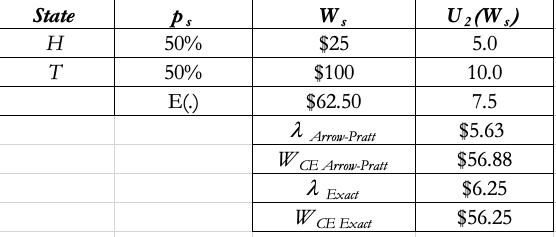# Mea Culpa concerning today’s Arrow-Pratt numeric illustration

I reexamined my analysis of the ad hoc exact and approximation methods from the review session today, and here is a screenshot from Excel of this problem:The fair coin toss involved payoffs of \$25 and \$100; the expected value of wealth under this coin toss is \$62.50, and the standard deviation is half of the dispersion between \$25 and \$100, or \$37.50.  Applying the “exact” method, we get a risk premium of \$6.25.  In calculating the risk premium under the Arrow-Pratt (approximation) method, I incorrectly calculated variance as 75^2 = 5,625.  Since variance is actually 37.5^2 = 1,406.25, the Arrow-Pratt equation produces a risk premium of \$5.63, not \$22.50.  I knew that right away that one of the model inputs had to be wrong, and it was the variance input, which was 4x the actual variance of the coin toss.